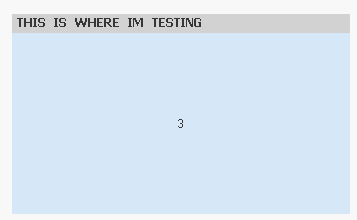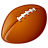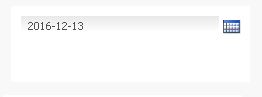# QlikView App Dev

Discussion Board for collaboration related to QlikView App Development.

cancel
Showing results for
Did you mean:Not applicable

## Problem with Set Analysis and date

Hi all,

I'm trying to build a calendar where I can select dates, and these dates should then change a graph for me.

How ever, what I ever do it just wont work... I'm quite new to Qlikview also, so knowing exactly the fault is difficult to find.
I've googled and search here and still can't figure it out.

Step one has been to make Calendar Objects linked to variables. Check and done. Simple.

The problem is when I'm trying to get the output in a graph.

This is the expression

=Sum({< LastTravelDate = {'>=(=Date(vStartDate))<=(=Date(vEndDate))'} >} NumOrders)

Even if I try and slim in down and fail search:

=Sum({< LastTravelDate = {=vPeriodStart1)} >} NumOrders)

Just wont work, the Variable turns gray, but my NumOrders wont go red...

1 Solution

Accepted SolutionsMVP

Is three the correct number?

=Sum({< LastTravelDate = {"\$(=vPeriodStart1)"}>} NumOrders)12 RepliesMVP

It would be difficult to know what might be missing without seeing a sample. Would you be able to provide one? In the mean time you can also read this blog

Dates in Set AnalysisMVP

Hi Kevin,

it would be a lot easier to troubleshoot if you could post a small sample app with your chart...

In the first expression, you should remove the single quotes within the braces:

=Sum({< LastTravelDate = {>=(=Date(vStartDate))<=(=Date(vEndDate))} >} NumOrders)

In the second expression, you don't need the equal sign for the variable, but you do need to enclose it in a \$-sign expansion and possibly convert to the date format:

=Sum({< LastTravelDate = {\$(vPeriodStart1)} >} NumOrders)

or

=Sum({< LastTravelDate = {\$(=date(vPeriodStart1))} >} NumOrders)

cheers,

Oleg TroyanskyCreator II

Kevin

As pointed out by others a sample app would make it easier to offer a solution. In the meantime you could always try entering your expression in a text box and playing around with the dates i.e.e selecting a small date range such as two or three days to make it easier to check the results.Not applicable
Author

Yes of course, stupid of me, forgetting the file.

I've attached it here.Not applicable
Author

Thank you for the reply, I have not attached a sample.

I was working around in a text box, as you mention, easier to check results... and without results..MVP

Is three the correct number?

=Sum({< LastTravelDate = {"\$(=vPeriodStart1)"}>} NumOrders)MVP

OK, much better now.

Your variable for Start Period contains a formula that returns a formatted Date. For that reason, it needs to be enclosed in a \$-sign expansion (because it's a variable) and in a set of single quotes (because it returns a formatted date. So, the corrected formula is:

=Sum({< LastTravelDate = {'\$(vPeriodStart1)'} >} NumOrders)

The corrected sample is also attached.

cheers,

Oleg TroyanskyNot applicable
Author

Hi!

Thank you for the help. I see the difference. But if I change the PeriodStart to vStartDate which is linked in the calendar, it wont function. Is there something wrong in the calendar ?MVP

I guess you just need to give it a proper format and it works:

=Sum({<LastTravelDate = {"\$(=date(vStartDate,'YYYY-MM-DD'))"}>} NumOrders)